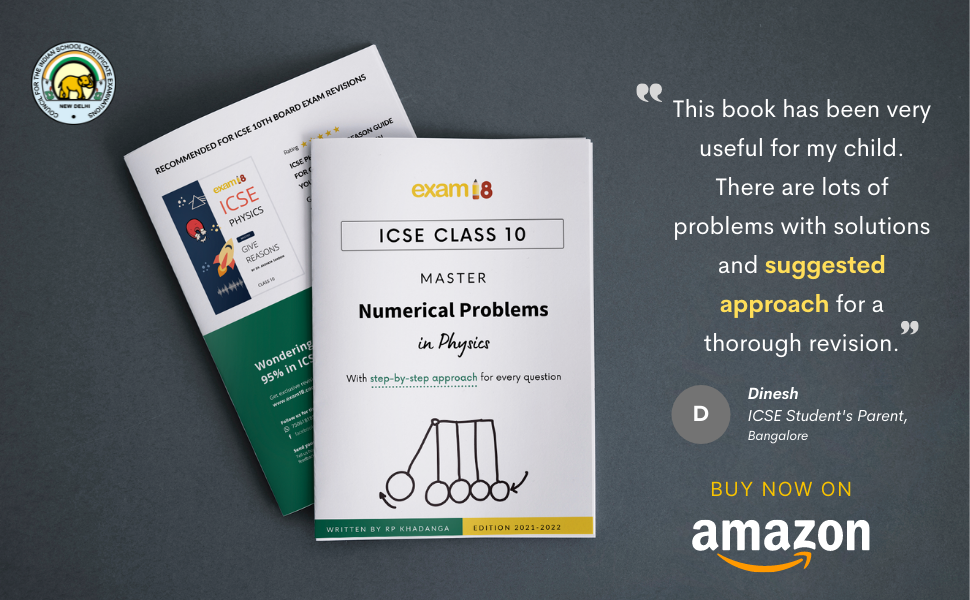# Exam18 ICSE Class 10 Master Physics Numerical Problems Guide (For 2022-23 Batch)

Regular price Rs. 500
Sale price Rs. 500 Regular price
91 students and teachers are ordering this book right now

🎁 Get Rs. 80 OFF with discount code ALMONDS

🚚 Free delivery across India on orders above Rs. 500

💰 Cash on delivery available

## Study Guide for Mastering Physics Numerical Problems

### For ICSE Class 10

Numerical questions play a large role in ICSE Physics exams, and many students lack a fundamental understanding of this subject, resulting in low marks. This special study guide teaches students how to approach numerical questions and solve them thoroughly.

Each chapter of this book includes:

• Step by step approach for every numerical problem
• Important formulas after every chapter
• Symbols and units
• Relationship between different formulas
• Important problems and solutions
• Suggested Approach to solve every numerical question
• Points to remember
• Detailed solution of every problem
• Explanation with diagrams and charts
• Specific hints

### Units Covered

#### (Strictly as per latest ICSE curriculum)

1. Force, Work, Power and Energy
2. Light
3. Sound
4. Electricity and Magnetism
5. Heat
6. Modern PhysicsICSE Class 10 Physics Master Numerical Problems is the one which will help you to get rid of your nightmares of solving Physics numerical problems. Students don't have to struggle about numerical problems and spend hours understanding it.

Based on the latest ICSE cirriculum (2022-23), this book will teach you how to solve practically any numerical question in your ICSE class 10 Physics exam.#### Each Chapeter includes

• Step by step approach for every numerical problem.
• Important formulas. (F=ma) after every chapter.
• Symbols and units (F= force, m= mass, a= acceleration)
• Relationship between different formulas.
• Important problems and solutions
• Suggested Approach to solve the numericals.
• Points to remember
• Detailed solution of every problem
• Diagram wise explanation
• Specific hints are also given.

### Chapters Covered

• Force, Work, Power and Energy
• Light
• Sound
• Electricity and Magnetism
• Heat
• Modern Physics

### In Summary

 SUBJECT Physics TARGET EXAM ICSE Class 10 EDITION 2023 AUTHOR RP Khadanga PAGES 132 PUBLISH DATE 2022-04-15 PUBLISHER Exam18 TYPE OF MATERIAL Printed Book/Paperback RETURN PERIOD 7 days